Maths-
General
Easy

Question

# Let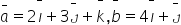and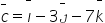A vector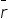is such that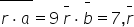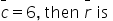##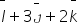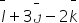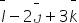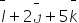Hint:

## The correct answer is:### The three vectors are as follows: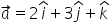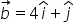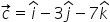Let the fourth vector be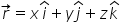We will write the dot products now: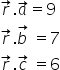We will take the dot products of the given vectors with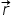.Now we have three simultaneous equations. We will solve them to get final answer.Step 1: Add the three equation.2x + 3y + z = 9+        4x + y           = 7 +        x - 3y - 7z    = 6_________________________________            7x + y - 6z  =  22                    ...(4)Step 2:  Subtract (2) from (4)7x + y - 6z = 22-          (-)4x +(-) y       = 7_________________________________3x - 6z    = 15Divide both the sides by 3.x - 2z = 5       ...(5)Step 3: Multiply equation (1) by 2 and add equation (5) to it.2(2x + 3y + z) = 2(9)4x + 6y + 2z  = 18+         x +  0  - 2z  = 5______________________________5x + 6y = 23            ...(6)Step 4: Multiply equation (2) by -6 and add (6) and (2)-6(4x + y) = -6(7)-24x - 6y = -42+            5x + 6y =  23__________________________________-19x + 0 = -19x = 1Step 5: Substituting value of x in equation (2) and (5)4(1) + y = 7y = 3(1) -2z = 5-2z = 4z = -2Substituting the values of x,y and z we get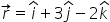This is the final answer.

We can solve simultaneous equations as we feel easy for us. There are not any particular steps to be followed. We just have to solve the equation to find the values of the variables. We try to eliminate the variables using addition and subtraction to single out single variable.

### Related Questions to study#### With Turito Foundation.#### Get an Expert Advice From Turito.